NEET  >  NEET Previous Year Questions: Some Basic Concepts Of Chemistry

# NEET Previous Year Questions: Some Basic Concepts Of Chemistry

Test Description

## 20 Questions MCQ Test Chemistry Class 11 | NEET Previous Year Questions: Some Basic Concepts Of Chemistry

NEET Previous Year Questions: Some Basic Concepts Of Chemistry for NEET 2023 is part of Chemistry Class 11 preparation. The NEET Previous Year Questions: Some Basic Concepts Of Chemistry questions and answers have been prepared according to the NEET exam syllabus.The NEET Previous Year Questions: Some Basic Concepts Of Chemistry MCQs are made for NEET 2023 Exam. Find important definitions, questions, notes, meanings, examples, exercises, MCQs and online tests for NEET Previous Year Questions: Some Basic Concepts Of Chemistry below.
Solutions of NEET Previous Year Questions: Some Basic Concepts Of Chemistry questions in English are available as part of our Chemistry Class 11 for NEET & NEET Previous Year Questions: Some Basic Concepts Of Chemistry solutions in Hindi for Chemistry Class 11 course. Download more important topics, notes, lectures and mock test series for NEET Exam by signing up for free. Attempt NEET Previous Year Questions: Some Basic Concepts Of Chemistry | 20 questions in 20 minutes | Mock test for NEET preparation | Free important questions MCQ to study Chemistry Class 11 for NEET Exam | Download free PDF with solutions
 1 Crore+ students have signed up on EduRev. Have you?
NEET Previous Year Questions: Some Basic Concepts Of Chemistry - Question 1

### 20 ml of decinormal solution of NaOH neutralises 25 ml of a solution of dibasic acid containing 3g. of the acid per 500 ml. The Molecular weight of the acid is

Detailed Solution for NEET Previous Year Questions: Some Basic Concepts Of Chemistry - Question 1

NaOH = dibasic acid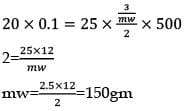NEET Previous Year Questions: Some Basic Concepts Of Chemistry - Question 2

### In a compound C, H and N are present in the ratio 9: 1: 3.5 by weight. Molecular mass of the compound is 108. Molecular formula of the compound is:

Detailed Solution for NEET Previous Year Questions: Some Basic Concepts Of Chemistry - Question 2

C% = 9/12 = 0.75 = 0.75/0.25 = 3
H% 1/1 =1 = 1/0.25 = 4

N= 3.5/14 = 0.25 = 0.25/0.25 = 1
Empirical formula = C3H4N
36+4+14=54
n = 108/54 = 2
(C3H4N) × 2
= C6H8N2

NEET Previous Year Questions: Some Basic Concepts Of Chemistry - Question 3

### Number of atoms is 560 g of Fe (atomic mass = 56 g mol-1)

Detailed Solution for NEET Previous Year Questions: Some Basic Concepts Of Chemistry - Question 3

Fe atom= 560/56 =10 mole
= 10 x NA atom
option(A) = 5 mol
5 x NA
option (B) = 20/1 = 20
20 x NA

NEET Previous Year Questions: Some Basic Concepts Of Chemistry - Question 4

What volume of hydrogen gas at 273 K and 1 atm pressure will be consumed in obtaining 21.6 g elemental boron (atomic mass = 10.8) from the reduction of  boron trichloride by hydrogen?

Detailed Solution for NEET Previous Year Questions: Some Basic Concepts Of Chemistry - Question 4

BCl3 + 3H ⇋ B+3HCl
BCl3 + 3/2 → B + 3HCl
3/2 mol → 1 mol
1 mol B → 3/2 molH2
2 mole → 3/2  x 2 = 6/2 = 3 mole
1 mole = 22.4 liter S.T.P
3----?
= 22.4 x 3
=67.2 litre

NEET Previous Year Questions: Some Basic Concepts Of Chemistry - Question 5

One mole of magnesium nitride on reaction with an excess of water gives

Detailed Solution for NEET Previous Year Questions: Some Basic Concepts Of Chemistry - Question 5

Mg3N+ 6H2O→ 3Mg(OH) 2 + 2NH3

NEET Previous Year Questions: Some Basic Concepts Of Chemistry - Question 6

How many moles of magnesium phosphate, Mg3 (PO4)2 will contain 0.25 mole of oxygen atoms?

Detailed Solution for NEET Previous Year Questions: Some Basic Concepts Of Chemistry - Question 6

Mg3(P04)→ 3Mg
→2 P
→ 8 mole oxygen
8 mole of oxygen - 1 mole Mg3 (P0)2
0.25 - ? 0.25/8 = 3 x 10-2

NEET Previous Year Questions: Some Basic Concepts Of Chemistry - Question 7

In the reaction, 2Al (s) + 6HCl (aq) → 2Al3+ (aq) + 6Cl- (aq) + 3H2(g)

Detailed Solution for NEET Previous Year Questions: Some Basic Concepts Of Chemistry - Question 7

2AI + 6HCl  →  2A l3+ + 6Cl- + 3H2
6 ----- 3 mol
1 mol ---- ?
3/6 = 1/2
= 0.5
At S.T.P= 22.4 - 1 mol
11.2 L

NEET Previous Year Questions: Some Basic Concepts Of Chemistry - Question 8

Volume occupied by one molecule of water (density = 1 g cm-3) is

Detailed Solution for NEET Previous Year Questions: Some Basic Concepts Of Chemistry - Question 8

Given

Density of water (ρ) = 1g/cm3

Molar mass of water (M) = 18 g/mol

∴ Molar volume (Vm) = M/ρ

Vm = 18 cm3/mol

∴ Volume of 1 molecule of water = Vm/NA

Volume of 1 molecule of water = 18/(6.022 × 1023)

Volume of 1 molecule of water = 2.989 × 10-23 ≈ 3 × 10-23.

NEET Previous Year Questions: Some Basic Concepts Of Chemistry - Question 9

How many moles of lead (II) chloride will be formed from a reaction between 6.5 g of Pbo and 3.2 g of HCl?

Detailed Solution for NEET Previous Year Questions: Some Basic Concepts Of Chemistry - Question 9

PbO + 2HCl
6.5\223
= 2.9 x 10-2
= 2.9x10-2 - x
nx = 9a
PbCl2 + H2O
3.2/36.5
= 8.76 x 10-2
8.76 x 10-2 - x
x = 2.9 x 10-2

NEET Previous Year Questions: Some Basic Concepts Of Chemistry - Question 10

100 ml of PH3 on heating forms P and H2. The volume change in the reaction is

Detailed Solution for NEET Previous Year Questions: Some Basic Concepts Of Chemistry - Question 10

PH⇋ P5 + 3/2 H2
1 mol 1+ 3/2 =2.5 mol
⟹ 100ml - 3/2 = 150ml
⟹150 - 100 = 50ml

NEET Previous Year Questions: Some Basic Concepts Of Chemistry - Question 11

Excess of carbon dioxide is passed through 50 mL of 0.5 M calcium hydroxide solution. After the composition of the reaction, the solution was evaporated to dryness. The solid calcium carbonate was completely neutralized with 0.1 N hydrochloric acid. The volume of the hydrochloric acid required is___________

Detailed Solution for NEET Previous Year Questions: Some Basic Concepts Of Chemistry - Question 11

N1V1   =  N2V2
50 x 01 = 0.1 x Vml
Vm = 500ml

NEET Previous Year Questions: Some Basic Concepts Of Chemistry - Question 12

25.3 g of sodium carbonate, Na2CO3 is dissolved in enough water to make 250 mL of solution. If sodium carbonate dissociates completely, molar concentration of sodium ions, Na+ and carbonate ions,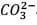are respectively. (At. mass of carbon = 40)

Detailed Solution for NEET Previous Year Questions: Some Basic Concepts Of Chemistry - Question 12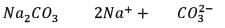= 2.53/106   2.59 × 10-2  5.9 × 10-2
0.238 × 0.25          = 1.18 × 10-3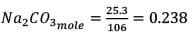250 ml - 0.238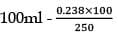= 0.955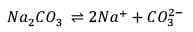0.955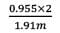0.955

NEET Previous Year Questions: Some Basic Concepts Of Chemistry - Question 13

The number of atoms in 0.1 mol of a triatomic gas is

Detailed Solution for NEET Previous Year Questions: Some Basic Concepts Of Chemistry - Question 13

Number of atoms = number of moles x Nx atomicity

= 0.1 x 6.02 x 1023 x 3

= 1.806 x 1023

NEET Previous Year Questions: Some Basic Concepts Of Chemistry - Question 14

How much time (in hours) would it take to distribute one Avogadro number of wheat grains if 1020 grains are distributed each second?

Detailed Solution for NEET Previous Year Questions: Some Basic Concepts Of Chemistry - Question 14

6 x 1023 gram wheat

1 sec → 102O gm distributed

1 hour - 3600sec - 1020 x 3600

= 3.6 x 1023 wheat

3.6 x 1023g  — 1 hour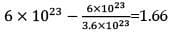NEET Previous Year Questions: Some Basic Concepts Of Chemistry - Question 15

For a reaction A + 2B → C, the amount of C formed by starting the reaction with 5 moles of A and 8 moles of B is

Detailed Solution for NEET Previous Year Questions: Some Basic Concepts Of Chemistry - Question 15

A +2B → C

t = 0  5    8    0

t= ?  5x  8-2x  x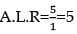nx = 9a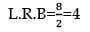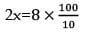x = 4
mole of C = 4 mole

NEET Previous Year Questions: Some Basic Concepts Of Chemistry - Question 16

A mixture of CaCl2 and NaCl weighing 4.44 g is treated with sodium carbonate solution to precipitate all the Ca2+ ions as calcium carbonate. The CaCO3 so obtained is heated strongly to get 0.56 g of CaO. The percentage of NaCl in the mixture is

Detailed Solution for NEET Previous Year Questions: Some Basic Concepts Of Chemistry - Question 16

CaCl2 + NaCl + NaC0→ CaC03
4.44     3.44
Co.C03milli  = CaCO3milli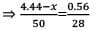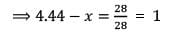x = 3.44
%purity= 3.44/4.44 x 100
=75%

NEET Previous Year Questions: Some Basic Concepts Of Chemistry - Question 17

If 1 mL of water contains 20 drops, then number of molecules in a drop of water is

Detailed Solution for NEET Previous Year Questions: Some Basic Concepts Of Chemistry - Question 17

1/18 = 5.5 x 10-2(mole of H20
20 drops - 5.5 x 10-2 mole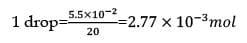= 2.77 x 10-3 x 6.023 x 1023
= 0.0167 x 1023
1.67 x 1021

NEET Previous Year Questions: Some Basic Concepts Of Chemistry - Question 18

In an experiment, 4 g of M2Ox oxide was reduced to 2.8g of the metal.Tf the atomic mass of the metal is 56 g mol-1, the number of O atoms in the oxide is

Detailed Solution for NEET Previous Year Questions: Some Basic Concepts Of Chemistry - Question 18

M20x → m
W1/E1 = W2/E2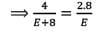⟹ 4E = 2.8E + 22.4
1.2E = 22.4
E= 22.4/1.2 = 18.6
Eq = mw/VF  = 18.6= 56/VF
M203    VF=3
The number of O atoms is 3

NEET Previous Year Questions: Some Basic Concepts Of Chemistry - Question 19

Which has the maximum number of molecules among the following?

Detailed Solution for NEET Previous Year Questions: Some Basic Concepts Of Chemistry - Question 19

1) 44/44 = 1 mole C02
2) 48/48 =1 mole
3) 8/2 = 4 mole H2
4) 64/64 =1 mole

NEET Previous Year Questions: Some Basic Concepts Of Chemistry - Question 20

The density of a solution prepared by dissolving 120 g of urea in 1000 g of water is 1.15 g/ml. The molarity of this solution is:

Detailed Solution for NEET Previous Year Questions: Some Basic Concepts Of Chemistry - Question 20

Mass of solute taken = 120g
Molecular mass of solute = 60u
Mass of solvent = 1000g
Density of solution = 1.15g/mL
Total mass of solution = 1000 + 120 = 1120g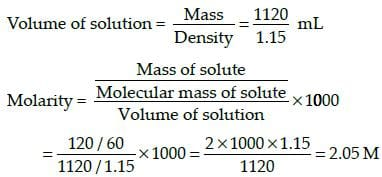## Chemistry Class 11

204 videos|331 docs|229 tests
 Use Code STAYHOME200 and get INR 200 additional OFF Use Coupon Code
Information about NEET Previous Year Questions: Some Basic Concepts Of Chemistry Page
In this test you can find the Exam questions for NEET Previous Year Questions: Some Basic Concepts Of Chemistry solved & explained in the simplest way possible. Besides giving Questions and answers for NEET Previous Year Questions: Some Basic Concepts Of Chemistry , EduRev gives you an ample number of Online tests for practice

## Chemistry Class 11

204 videos|331 docs|229 tests

### How to Prepare for NEET

Read our guide to prepare for NEET which is created by Toppers & the best Teachers